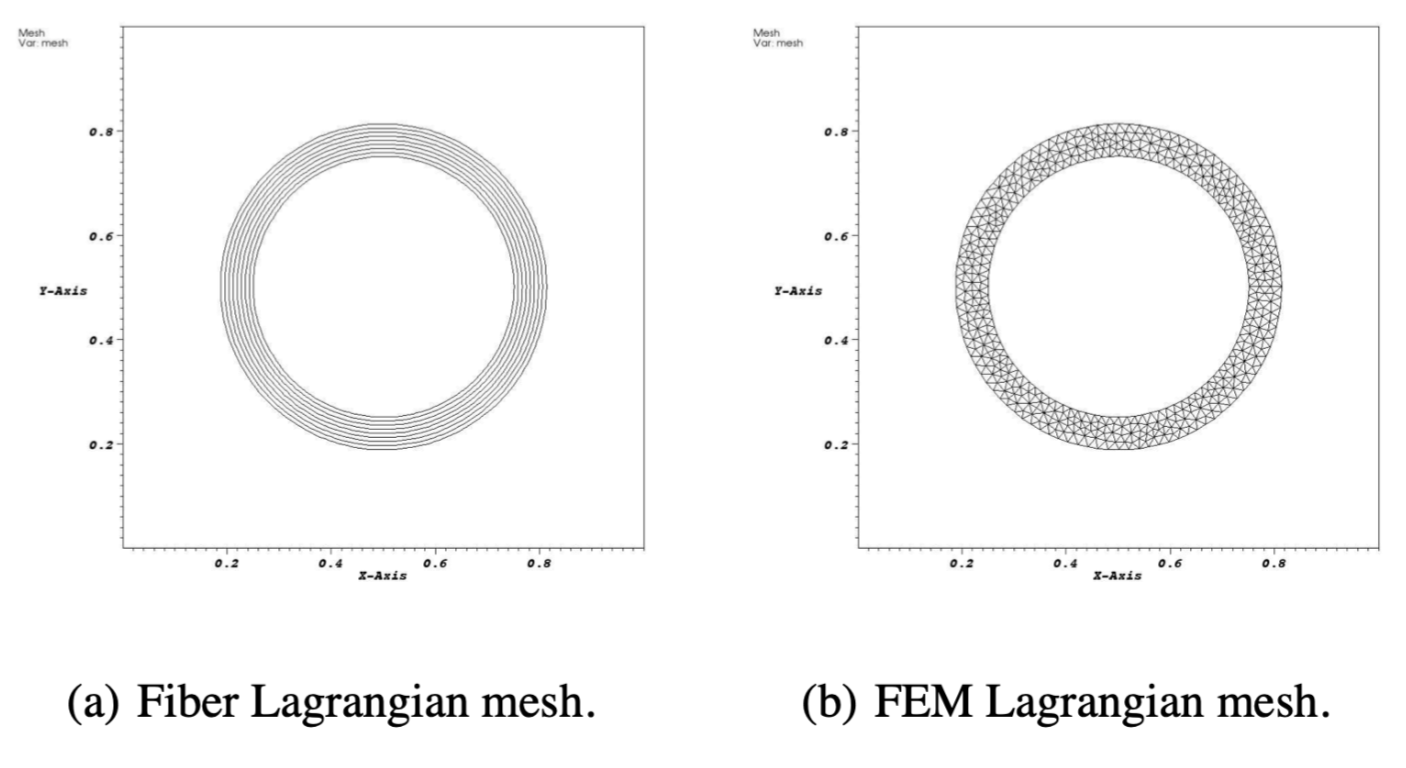## 正交异性壳体

1. 计算区域为 $\Omega=[0,1]\times[0,1]$ ，周期边界条件

2. 初始的固体区域用曲线坐标描述，

$\chi(\textbf{s},0)=\left(\;\;\begin{array}{ll} \text{cos}(s_{1}/R)(R+s_{2})+0.5 \\ \text{sin}(s_{1}/R)(R+\gamma+s_{2})+0.5 \end{array}\;\;\right)$

#### 参考构型中的中的固体力如何计算

$W^{\mathrm{e}}(\mathbb{F})=\frac{\mu^{\mathrm{e}}}{2 w}\left\|\frac{\partial \chi}{\partial s_1}\right\|^2=\frac{\mu^{\mathrm{e}}}{2 w} \mathbb{F}_{\alpha 1} \mathbb{F}_{\alpha 1}$

$\mathbb{P}^{\mathrm{e}}=\frac{\partial W^{\mathrm{e}}}{\partial \mathbb{F}}=\frac{\mu^{\mathrm{e}}}{w}\left(\begin{array}{cc} \frac{\partial \chi_1}{\partial s_1} & 0 \\ \frac{\partial \chi_2}{\partial s_1} & 0 \end{array}\right)=\frac{\mu^{\mathrm{e}}}{w}\left(\begin{array}{ll} \mathbb{F}_{11} & 0 \\ \mathbb{F}_{21} & 0 \end{array}\right)$

$\mathbf{G}=\frac{\mu}{w} \frac{\partial^2 \chi}{\partial s_1^2}=\frac{\mu}{w} \frac{1+s_2}{R}\left(\begin{array}{c} -\cos \left(s_1 / R\right) \\ -\sin \left(s_1 / R\right) \end{array}\right)=-\frac{\mu}{w} \frac{1+s_2}{R} \mathbf{r}$

$p(x, t) = \begin{cases} p_0 + {\frac{\mu^e}{R}} & r \leq R, \\ p_0 + {\frac{\mu^e}{w}\frac{R + w - r}{R}} & R < r \leq R + w, \\ p_0 & r > R + w. \end{cases}$

$p_0 = \frac{\pi \mu^e}{3w}{\left(R^2-\frac{ (R+w)^3}{R}\right)},$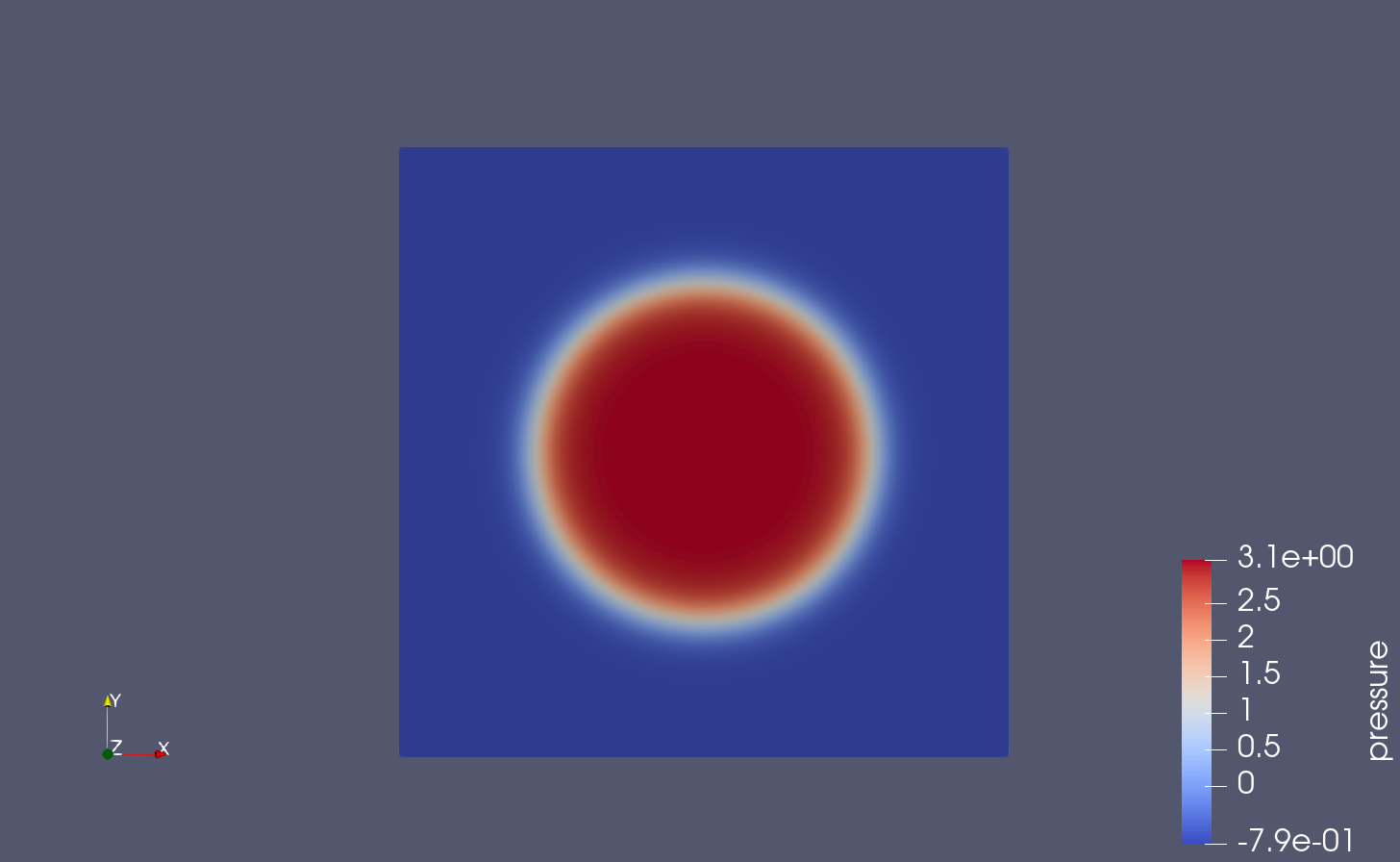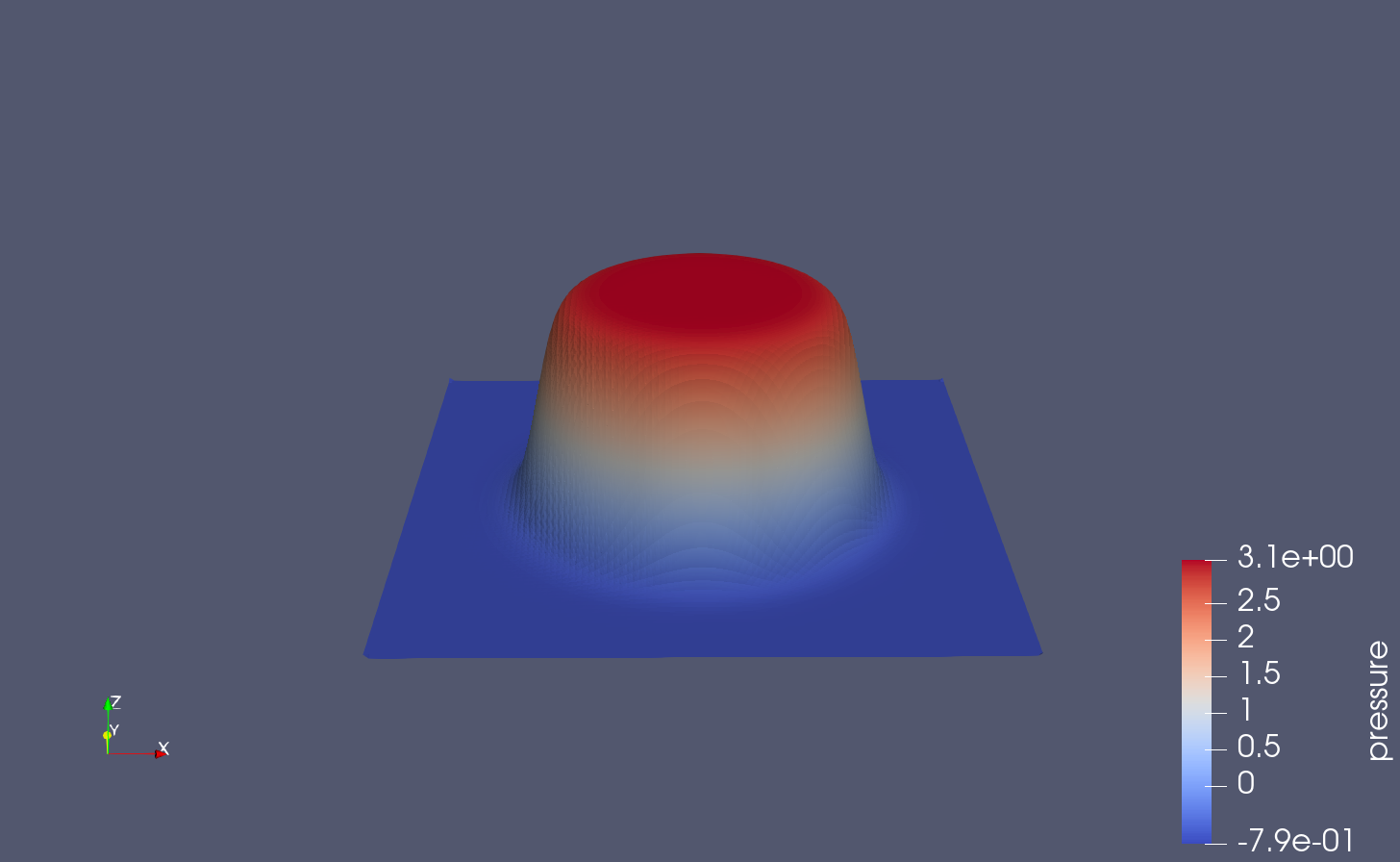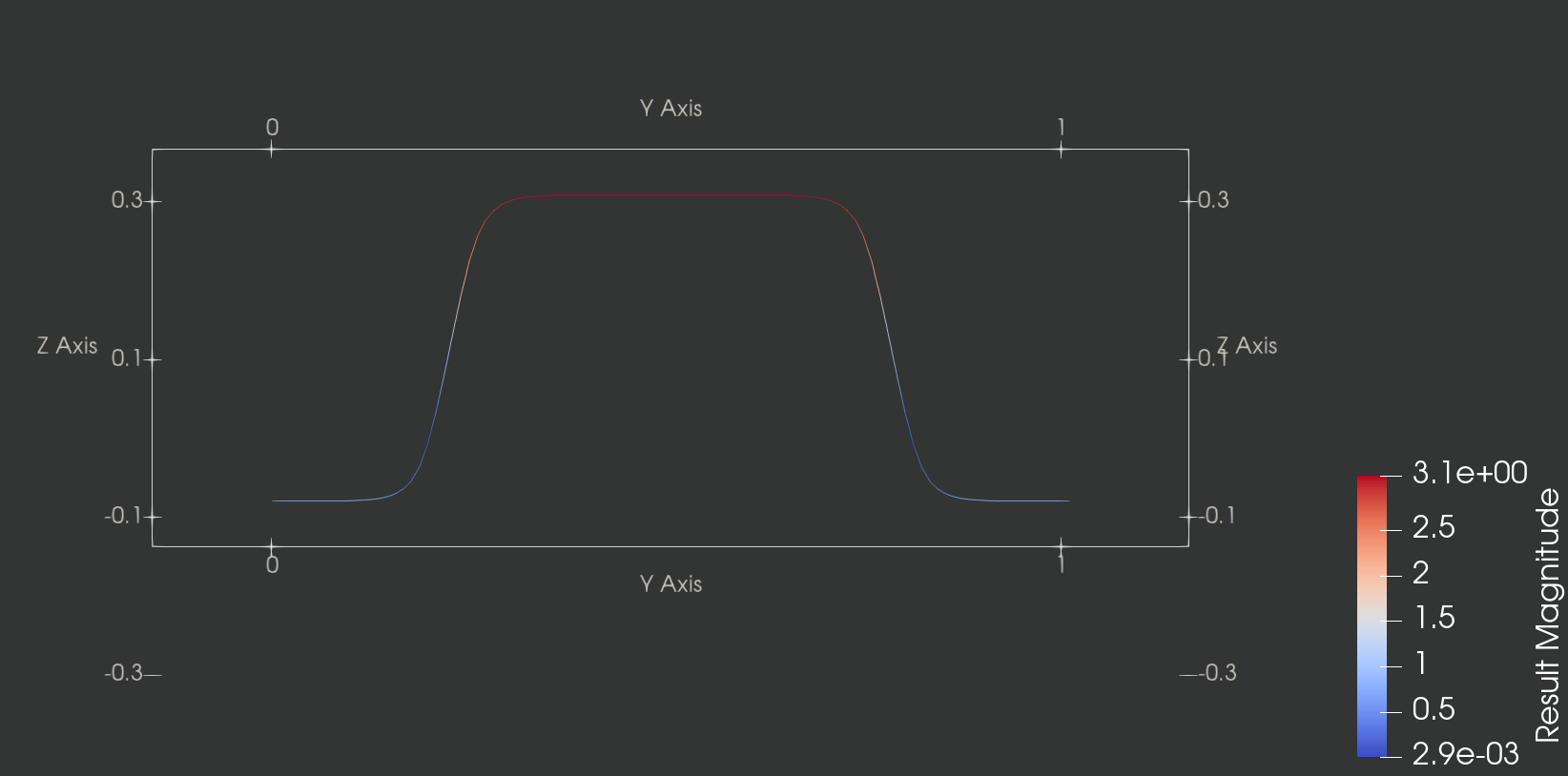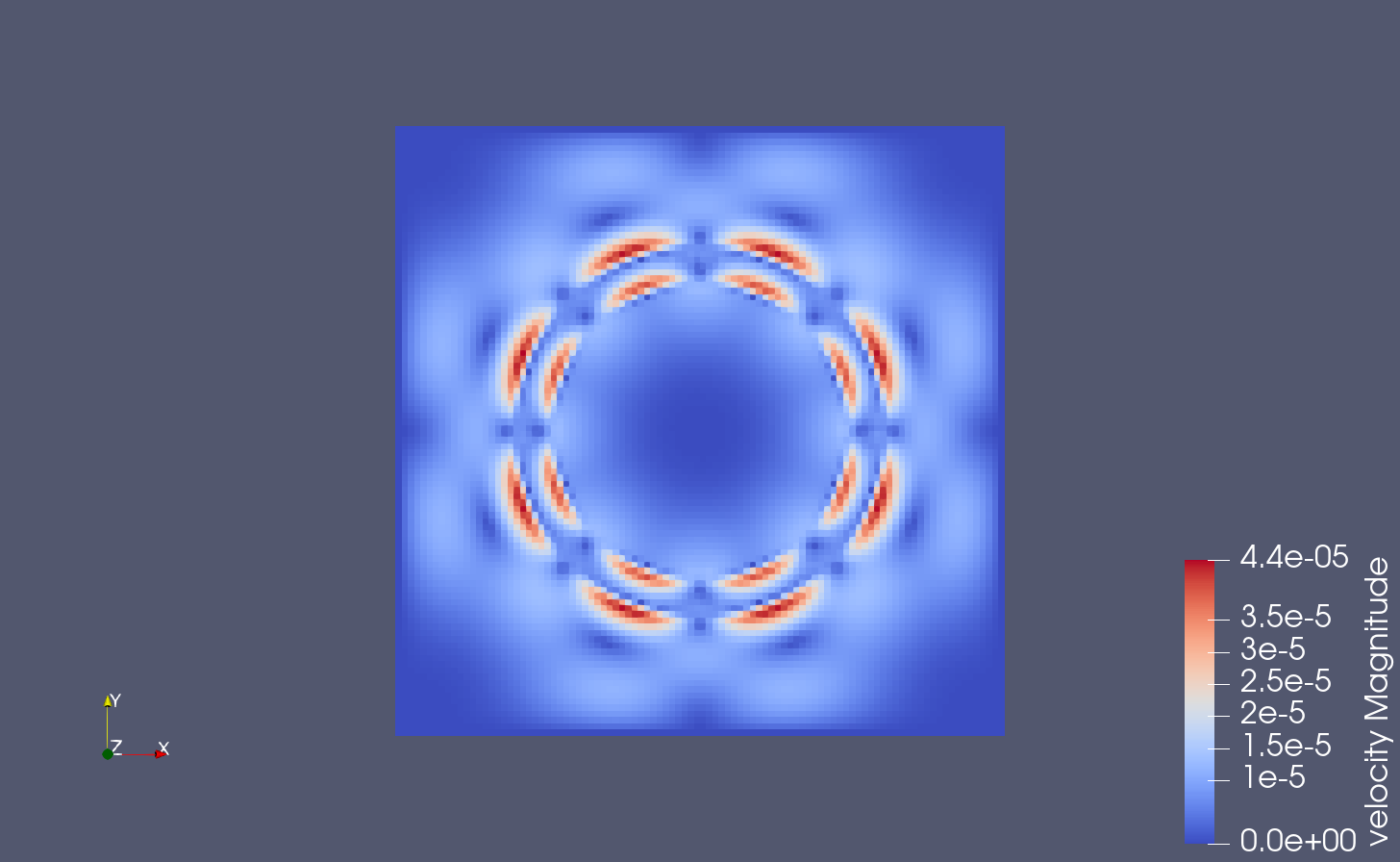$W(F)=\frac{\mu^{s}}{2w}I_{1}(\mathbb{C}),$

$\mathbb{P}=\frac{\mu^{s}}{w}\mathbb{F}.$

$\gamma=0$时, 结构体处于平衡状态，施加条件$\int_{\Omega}p(x,t)dx=0$，可得

$p(x,t)= \begin{cases}{l} p_{0}+\mu^{s}(\frac{1}{R}-\frac{1}{R+w}), &r\leq R,\\ p_{0}+\frac{\mu^{s}}{w}(\frac{1}{R}(R+w+r)+\frac{R}{R+w}) &R

### 画出收敛率折线图

1. 总共有五条线，三条为计算结果，两条斜率为1和2的参考线。
2. 三条计算结果分别为Mac=1, 2, 4的线。
3. 每条线上的节点分别对应背景网格64，128，256，512，1024五个数据。
4. 时间步长为0.24*dx。
5. 速度分别依L1, L2, L∞ 范数2阶收敛。
6. 压强分别依L1, L2, L∞ 范数2阶、1.5阶、1阶收敛。
7. $\rho=1,\mu=1,$$\mu^{e}=1$

$\rho=1,\mu=1,$$\mu^{e}=1$,我们考虑时间区间$0\leq t\leq 3$.图8总结了在时间$t=3$时，使用$M_{fac}=1,2,4$$\Delta t=0.25\Delta x$,$N=64,128,256,512$$1024$的误差数据.$u$的所有范数都可以观察到一阶收敛性。一阶收敛性也可以通过$p$$L^{1}$范数观到。$p$在此问题的流固耦合界面上是不连续的。然而当前的方法在$L^{2}$范数下产生的收敛速率是0.5和在$L^{\infty}$范数下是不收敛的。

1. 除了各向异性材料各向同性材料，还有正交异性材料正交异性材料指的是在三个互相垂直的方向上具有不同的性质的材料。心肌组织就是一种正交异性材料。 ↩︎

2. 文章中周期边界条件定义在区域 $\Omega$ 上的函数为周期函数。 ↩︎

3. 一般指定义在四边形网格、六边形网格上的一阶节点元为$Q1$元，定义在三角形、四面体单元上的节点元一般为P1元、P2元。 ↩︎